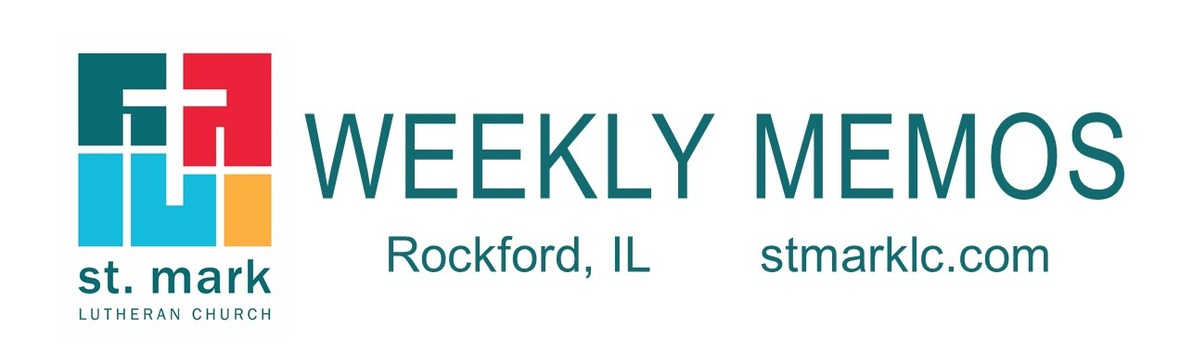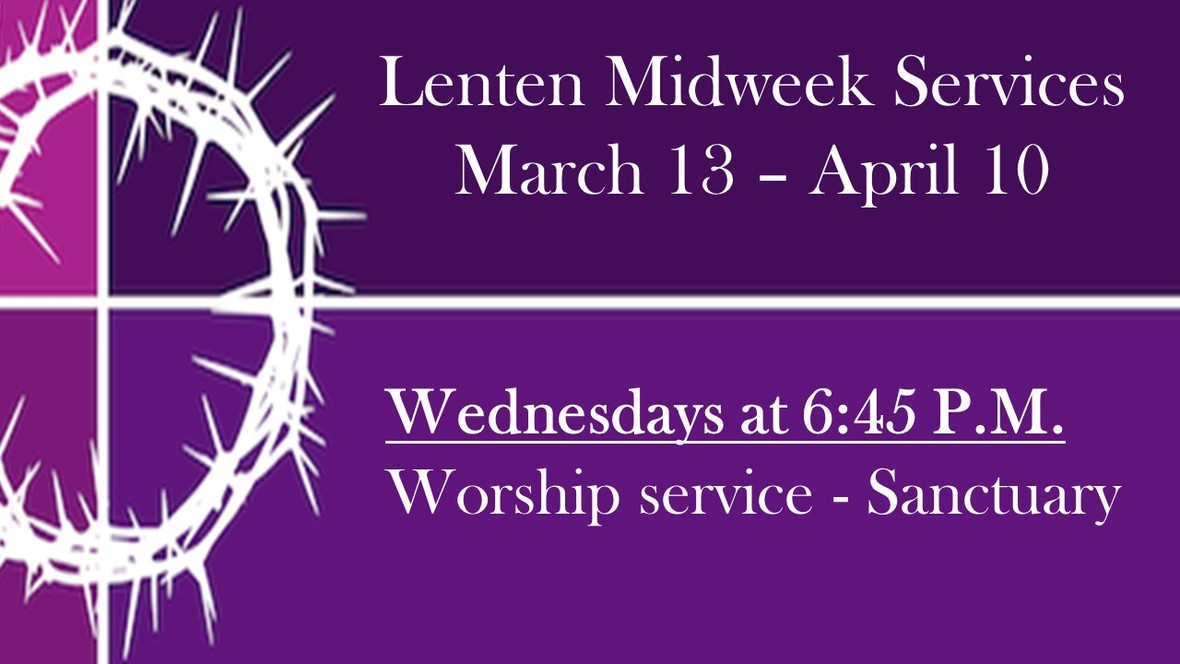Like   Tweet## Pastoral ReflectionPastor Mark's 2019 Sabbatical
Pastor Mark Hagen

In less than four months, I will embark on a much -anticipated sabbatical . . . read more.

 table div table+table+table+table div table{width:100%;padding:0}table div table+table+table+table div table img{width:96.23%;padding:0;float:none}table div table+table+table+table div table td{width:100%;padding:0 1.88% 18px}/* styles */## This Week at St. Mark March 17-24, 2019

 table div table+table+table+table+table+table div table{width:100%;padding:0}table div table+table+table+table+table+table div table img{width:96.23%;padding:0;float:none}table div table+table+table+table+table+table div table td{width:100%;padding:0 1.88% 18px}/* styles */## Sunday, March 17

8:00 A.M. Worship
9:30 A.M. Worship
9:30 A.M. Sunday School
10:45 A.M. New Members Orientation
11:00 A.M. Worship

## Monday, March 18

9:00 A.M. Classic Chorale Rehearses
4:30 P.M. MEMOS Deadline
6:30 P.M. Emotions Anonymous
6:30 P.M. Blue Lights
7:00 P.M. Theology Pub

## Tuesday, March 19

10:00 A.M. Staff Meeting
11:00 A.M. St. Mark Duplicate Bridge
6:30 P.M. Executive Meeting

## Wednesday, March 20

9:00 A.M. Women's Bible Study
9:00 A.M. Men's Bible Study
9:30 A.M. St. Mark Ringers
5:30 P.M. Good News Bearers
6:00 P.M. Bible Study
6:30 P.M. Confirmation
6:45 P.M. Lenten Worship Service
7:15 P.M. Voices of St. Mark

## Thursday, March 21

7:30 A.M. Panera Book Study
9:00 A.M. Cherry Valley Bridge Group
2:00 P.M. Barnabas Ministry
6:30 P.M. Emotions Anonymous
6:30 P.M. Diakonia
7:00 P.M. Praise Band Rehearses

## Saturday, March 23

6:00 P.M. Train Group

## Sunday, March 24

8:00 A.M. Worship
9:30 A.M. Worship
9:30 A.M. Baptism of Lucy Magdziarz
9:30 A.M. Sunday School
10:45 A.M. New Members Orientation
11:00 A.M. Worship

 table div table+table+table+table+table+table+table+table+table div table{width:100%;padding:0}table div table+table+table+table+table+table+table+table+table div table img{width:96.23%;padding:0;float:none}table div table+table+table+table+table+table+table+table+table div table td{width:100%;padding:0 1.88% 18px}/* styles */## News and Events

 table div table+table+table+table+table+table+table+table+table+table+table div table{width:100%;padding:0}table div table+table+table+table+table+table+table+table+table+table+table div table img{width:96.23%;padding:0;float:none}table div table+table+table+table+table+table+table+table+table+table+table div table td{width:100%;padding:0 1.88% 18px}/* styles *//* styles */ Mid-Week Lenten Service Our Wednesday evening services have begun. Our next Lenten service will be March 20 at 6:45 P.M. in the sanctuary. Our Lenten services will center on, "Five Gifts of Discipleship." Our scripture readings will come from Paul’s letter to the Philippians. I encourage you to read Philippians in advance as you prepare to join us for Wednesday evening worship this Lenten season. The topic for March 20 is: Hear the word of God and share in the Lord's supper. Philippians 3:7-11, lead by Pastor Chad.table div table+table+table+table+table+table+table+table+table+table+table+table+table+table div table{width:100%;padding:0}table div table+table+table+table+table+table+table+table+table+table+table+table+table+table div table img{width:96.23%;padding:0;float:none}table div table+table+table+table+table+table+table+table+table+table+table+table+table+table div table td{width:100%;padding:0 1.88% 18px}/* styles */Saturday, April 27, 9:00-Noon in the St. Mark Common Grounds Cafe. You are invited for activities, brunch, and conversation. Suggested donation \$5. Childcare will be available. Sign-up soon!

 table div table+table+table+table+table+table+table+table+table+table+table+table+table+table+table+table div table{width:100%;padding:0}table div table+table+table+table+table+table+table+table+table+table+table+table+table+table+table+table div table img{width:96.23%;padding:0;float:none}table div table+table+table+table+table+table+table+table+table+table+table+table+table+table+table+table div table td{width:100%;padding:0 1.88% 18px}/* styles */Lenten Devotionals
Free Lenten devotional booklets are available on the name tag tables in the narthex. This year’s devotions are entitled Thy Will Be Done by Ted Schroeder.

 table div table+table+table+table+table+table+table+table+table+table+table+table+table+table+table+table+table+table div table{width:100%;padding:0}table div table+table+table+table+table+table+table+table+table+table+table+table+table+table+table+table+table+table div table img{width:96.23%;padding:0;float:none}table div table+table+table+table+table+table+table+table+table+table+table+table+table+table+table+table+table+table div table td{width:100%;padding:0 1.88% 18px}/* styles */Miss a Sunday at St. Mark? Did you know that you can watch the sermons online? Click here to watch.

 table div table+table+table+table+table+table+table+table+table+table+table+table+table+table+table+table+table+table+table+table div table{width:100%;padding:0}table div table+table+table+table+table+table+table+table+table+table+table+table+table+table+table+table+table+table+table+table div table img{width:96.23%;padding:0;float:none}table div table+table+table+table+table+table+table+table+table+table+table+table+table+table+table+table+table+table+table+table div table td{width:100%;padding:0 1.88% 18px}/* styles */Sign-up for an Ice Hogs Game
St. Mark is going to the game on Sunday, March 31, 4:00 P.M. game. Tickets are \$22. This game will honor the Greg Lindmark foundation. There will be autographs with Chris Chelios Join in on the fun! Please, sign-up in Fellowship Hall.

 table div table+table+table+table+table+table+table+table+table+table+table+table+table+table+table+table+table+table+table+table+table+table div table{width:100%;padding:0}table div table+table+table+table+table+table+table+table+table+table+table+table+table+table+table+table+table+table+table+table+table+table div table img{width:96.23%;padding:0;float:none}table div table+table+table+table+table+table+table+table+table+table+table+table+table+table+table+table+table+table+table+table+table+table div table td{width:100%;padding:0 1.88% 18px}/* styles */New Member Orientation
The spring New Member Orientation dates are March 17 - 24 from 10:45-11:45 A.M. Please contact Pastor Chad McKenna at prmckenna@stmarklc.com if you are interested in becoming a member of St. Mark. We will receive new members on March 31 at the 9:30 A.M. service.

 table div table+table+table+table+table+table+table+table+table+table+table+table+table+table+table+table+table+table+table+table+table+table+table+table div table{width:100%;padding:0}table div table+table+table+table+table+table+table+table+table+table+table+table+table+table+table+table+table+table+table+table+table+table+table+table div table img{width:96.23%;padding:0;float:none}table div table+table+table+table+table+table+table+table+table+table+table+table+table+table+table+table+table+table+table+table+table+table+table+table div table td{width:100%;padding:0 1.88% 18px}/* styles */Theology Pub
Join Pastor Chad for a pint and great conversation at Carlyle Brewing Co. (215 East State St.) on Monday, March 18 at 7:00 P.M.

 table div table+table+table+table+table+table+table+table+table+table+table+table+table+table+table+table+table+table+table+table+table+table+table+table+table+table div table{width:100%;padding:0}table div table+table+table+table+table+table+table+table+table+table+table+table+table+table+table+table+table+table+table+table+table+table+table+table+table+table div table img{width:96.23%;padding:0;float:none}table div table+table+table+table+table+table+table+table+table+table+table+table+table+table+table+table+table+table+table+table+table+table+table+table+table+table div table td{width:100%;padding:0 1.88% 18px}/* styles */Lenten Books
Our St. Mark Library has a display of books, especially for Lent. Stop in. The selection includes devotional guides, books on the life of Jesus, inspirational items, and more.

 table div table+table+table+table+table+table+table+table+table+table+table+table+table+table+table+table+table+table+table+table+table+table+table+table+table+table+table+table div table{width:100%;padding:0}table div table+table+table+table+table+table+table+table+table+table+table+table+table+table+table+table+table+table+table+table+table+table+table+table+table+table+table+table div table img{width:96.23%;padding:0;float:none}table div table+table+table+table+table+table+table+table+table+table+table+table+table+table+table+table+table+table+table+table+table+table+table+table+table+table+table+table div table td{width:100%;padding:0 1.88% 18px}/* styles */Sheri Anderson will be celebrating her 80th birthday, March 20! Birthday blessings from your St. Mark family.

 table div table+table+table+table+table+table+table+table+table+table+table+table+table+table+table+table+table+table+table+table+table+table+table+table+table+table+table+table+table+table div table{width:100%;padding:0}table div table+table+table+table+table+table+table+table+table+table+table+table+table+table+table+table+table+table+table+table+table+table+table+table+table+table+table+table+table+table div table img{width:96.23%;padding:0;float:none}table div table+table+table+table+table+table+table+table+table+table+table+table+table+table+table+table+table+table+table+table+table+table+table+table+table+table+table+table+table+table div table td{width:100%;padding:0 1.88% 18px}/* styles */## Youth MinistrySt. Mark High School Youth High Adventure Trip - We have four spots available!
Apostle Islands High Adventure Trip June 23-28th 2019. Cost is \$500 and includes all food, lodging, kayak, etc. Please see Melinda Alekna if you have questions and/or want to sign up. Check out the web site www.Adv-Vac.com.

 table div table+table+table+table+table+table+table+table+table+table+table+table+table+table+table+table+table+table+table+table+table+table+table+table+table+table+table+table+table+table+table+table+table div table{width:100%;padding:0}table div table+table+table+table+table+table+table+table+table+table+table+table+table+table+table+table+table+table+table+table+table+table+table+table+table+table+table+table+table+table+table+table+table div table img{width:96.23%;padding:0;float:none}table div table+table+table+table+table+table+table+table+table+table+table+table+table+table+table+table+table+table+table+table+table+table+table+table+table+table+table+table+table+table+table+table+table div table td{width:100%;padding:0 1.88% 18px}/* styles */## Children's MinistrySunday School will be running a Lenten Giving Project, starting next Sunday. For the six weeks of Lent, all of the money collected for Sunday School offering will be donated to Project Undershare, an organization that gives new underwear and socks to children in Rockford who need them.

 table div table+table+table+table+table+table+table+table+table+table+table+table+table+table+table+table+table+table+table+table+table+table+table+table+table+table+table+table+table+table+table+table+table+table+table+table div table{width:100%;padding:0}table div table+table+table+table+table+table+table+table+table+table+table+table+table+table+table+table+table+table+table+table+table+table+table+table+table+table+table+table+table+table+table+table+table+table+table+table div table img{width:96.23%;padding:0;float:none}table div table+table+table+table+table+table+table+table+table+table+table+table+table+table+table+table+table+table+table+table+table+table+table+table+table+table+table+table+table+table+table+table+table+table+table+table div table td{width:100%;padding:0 1.88% 18px}/* styles */## Outreach MinistryThe Outreach Committee is asking members to donate new socks during Lent. The Rockford Public Schools identified the need for socks for the children. Shop all sizes, all colors, and load up the Red Truck in Fellowship Hall! Thanks for your help with this project.

 table div table+table+table+table+table+table+table+table+table+table+table+table+table+table+table+table+table+table+table+table+table+table+table+table+table+table+table+table+table+table+table+table+table+table+table+table+table+table+table div table{width:100%;padding:0}table div table+table+table+table+table+table+table+table+table+table+table+table+table+table+table+table+table+table+table+table+table+table+table+table+table+table+table+table+table+table+table+table+table+table+table+table+table+table+table div table img{width:96.23%;padding:0;float:none}table div table+table+table+table+table+table+table+table+table+table+table+table+table+table+table+table+table+table+table+table+table+table+table+table+table+table+table+table+table+table+table+table+table+table+table+table+table+table+table div table td{width:100%;padding:0 1.88% 18px}/* styles */Dick Zimmanck is moving to Indiana to be closer to family. His new address is
3802 S. Sare Rd., #202
Bloomington, IN 47401
His last Sunday in church will be March 17.

 table div table+table+table+table+table+table+table+table+table+table+table+table+table+table+table+table+table+table+table+table+table+table+table+table+table+table+table+table+table+table+table+table+table+table+table+table+table+table+table+table+table div table{width:100%;padding:0}table div table+table+table+table+table+table+table+table+table+table+table+table+table+table+table+table+table+table+table+table+table+table+table+table+table+table+table+table+table+table+table+table+table+table+table+table+table+table+table+table+table div table img{width:96.23%;padding:0;float:none}table div table+table+table+table+table+table+table+table+table+table+table+table+table+table+table+table+table+table+table+table+table+table+table+table+table+table+table+table+table+table+table+table+table+table+table+table+table+table+table+table+table div table td{width:100%;padding:0 1.88% 18px}/* styles */table div table+table+table+table+table+table+table+table+table+table+table+table+table+table+table+table+table+table+table+table+table+table+table+table+table+table+table+table+table+table+table+table+table+table+table+table+table+table+table+table+table+table+table div table{width:100%;padding:0}table div table+table+table+table+table+table+table+table+table+table+table+table+table+table+table+table+table+table+table+table+table+table+table+table+table+table+table+table+table+table+table+table+table+table+table+table+table+table+table+table+table+table+table div table img{width:96.23%;padding:0;float:none}table div table+table+table+table+table+table+table+table+table+table+table+table+table+table+table+table+table+table+table+table+table+table+table+table+table+table+table+table+table+table+table+table+table+table+table+table+table+table+table+table+table+table+table div table td{width:100%;padding:0 1.88% 18px}/* styles */## Our St. Mark members:

Kevin Cotter, Inara McGroarty, Marsha Carlson, Steve Carter, Dick Zimmanck, David Young, Russ Oellig, Linda Swanson, Pam Cottrill, Keith Haggestad, Naomi Hagen.

## Family and friends of St. Mark members:

Mary Graham, Doug Nelson, Barbara, Heckart, Tom Wolfe, Kim Menke, Noah and Ellie Adams, Jameson Elder, Norma Comstock, Butch Cotter, Scott Jones, Ethan Whitney, Carolyn King, Tom Severson, Katie Butera, Ashton Henley, Nathan Gyllin.

## Those serving in the military and their families:

Bailey Panjkovich, Xander Gehrke, Jim Tammen, Brandon Ballenger, Taylor Eversole, Dean Barron.

 table div table+table+table+table+table+table+table+table+table+table+table+table+table+table+table+table+table+table+table+table+table+table+table+table+table+table+table+table+table+table+table+table+table+table+table+table+table+table+table+table+table+table+table+table+table+table div table{width:100%;padding:0}table div table+table+table+table+table+table+table+table+table+table+table+table+table+table+table+table+table+table+table+table+table+table+table+table+table+table+table+table+table+table+table+table+table+table+table+table+table+table+table+table+table+table+table+table+table+table div table img{width:96.23%;padding:0;float:none}table div table+table+table+table+table+table+table+table+table+table+table+table+table+table+table+table+table+table+table+table+table+table+table+table+table+table+table+table+table+table+table+table+table+table+table+table+table+table+table+table+table+table+table+table+table+table div table td{width:100%;padding:0 1.88% 18px}/* styles *//* styles */ Office Hours Monday - Friday, 8:00 A.M. - 4:00 P.M. Telephone: 815-398-3557 Publications St. Mark Memos, the weekly e-newsletter, is distributed each Thursday. The deadline for submission is Monday by 4:00 P.M. Please click to send submissions. Sermons Online Miss a Sunday at St. Mark? Please visit our website and watch the sermons online. Please click to watch.